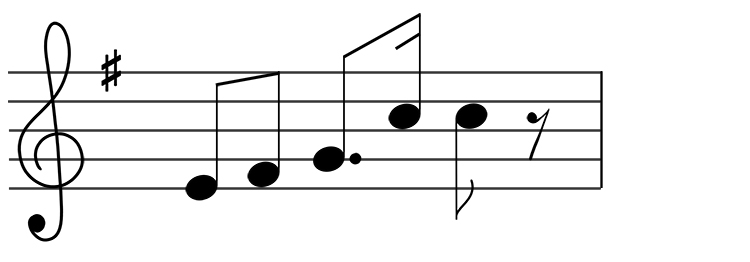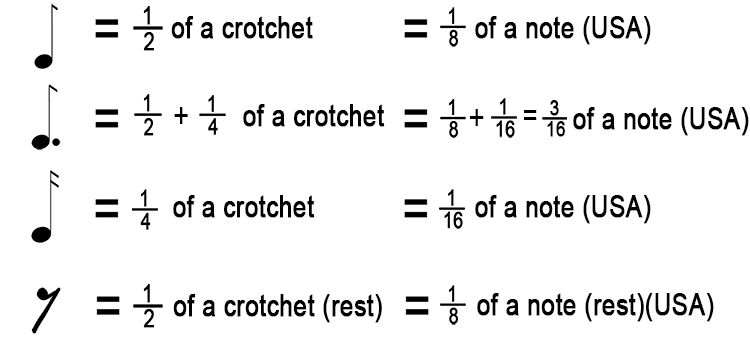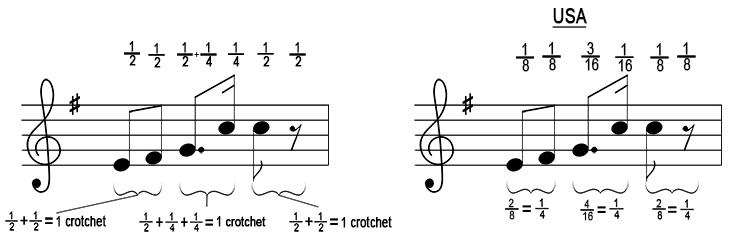# Time Signatures Exam theory questions - example 3

What is the correct time signature?In order to do this you need to break down the value of each note:Then write these values above each note on the bar:There are 3 crotchets in this bar, so the time signature would be:

3/4 = 3  Ncirctimes crotchets = 3  Ncirctimes1/4 notes (USA)

The time signature is 3/4